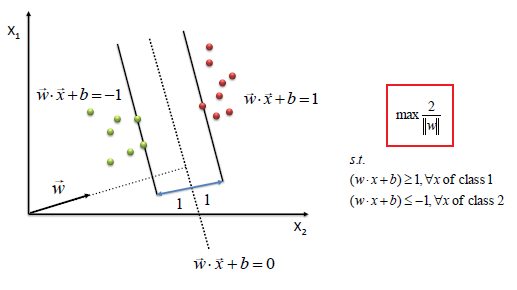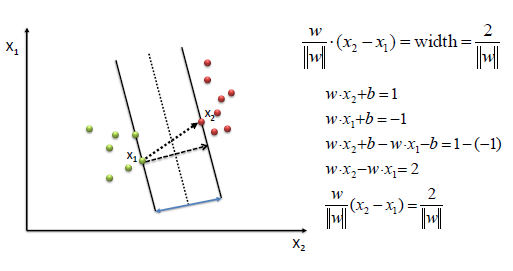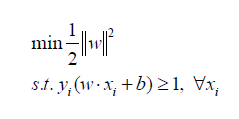2 views

From what I've seen, seems like the separation hyperplane must be in the form

x.w + b = 0.

I don't get very well this notation. From what I understand, x.w is the inner product, so its the result will be a scalar. How can be that you can represent a hyperplane by a scalar + b? I'm quite confused.

Also, even if it was x + b = 0, wouldn't it be of a hyperplane that passes right through the origin? From what I understand a separating hyperplane doesn't always pass through the origin!

by (108k points)
edited by

SVM performs classification by finding the hyperplane(a subspace whose dimension is one less than that of its surrounding space) that maximizes the margin between the two classes. The hyperplane that defines the cases is known as the support vectors.

Algorithm

1. Define an optimal hyperplane: maximize the margin

2. Extend the above definition for non-linearly separable problems: have a penalty term for misclassifications.

3. Do the mapping of the data to the top space so that it is easier to classify with linear decision levels: reformulate the problem so that data is mapped completely to this space.

To define an optimal hyperplane we need to maximize the width of the margin (w).We find w and b by solving the following objective function using Quadratic Programming.Coming to your first question, the value of b will not scalar as it will be decided by the above equation.

The beauty of SVM is that if the data is linearly separable, there is a unique global minimum value. An ideal SVM analysis should produce a hyperplane that completely separates the vectors (cases) into two non-overlapping classes. However, perfect separation may not be possible, or it may result in a model with so many cases that the model does not classify correctly. In this situation, SVM finds the hyperplane that maximizes the margin and minimizes the misclassifications.

If you want know about Artificial Intelligence and Deep Learning then you can watch this video: